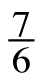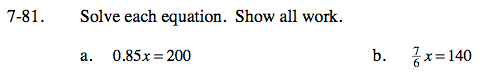Home > CC2MN > Chapter cc27 > Lesson cc27.1.7 > Problem7-81

7-81.
1. Solve each equation. Show all work. Homework Help ✎

1. 0.85x = 200

2.x = 140Divide both sides of the equation by 0.85 to isolate x.

$\frac{0.85\textit{x}}{0.85}=\frac{200}{0.85}$

x = 235.29

$\text{Multiply each side by }\frac{6}{7}\text{ to isolate }x.$